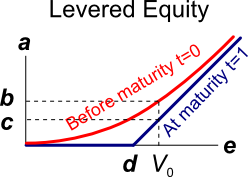# Fight Finance

#### CoursesTagsRandomAllRecentScores

In the Merton model of corporate debt, buying a levered company's debt is equivalent to buying risk free government bonds and:

In the Merton model of corporate debt, buying a levered company's shares is equivalent to:

In the Merton model of corporate debt, buying a levered company's debt is equivalent to buying the company's assets and:

A risky firm will last for one period only (t=0 to 1), then it will be liquidated. So it's assets will be sold and the debt holders and equity holders will be paid out in that order. The firm has the following quantities:

$V$ = Market value of assets.

$E$ = Market value of (levered) equity.

$D$ = Market value of zero coupon bonds.

$F_1$ = Total face value of zero coupon bonds which is promised to be paid in one year.The levered equity graph above contains bold labels a to e. Which of the following statements about those labels is NOT correct?

A risky firm will last for one period only (t=0 to 1), then it will be liquidated. So it's assets will be sold and the debt holders and equity holders will be paid out in that order. The firm has the following quantities:

$V$ = Market value of assets.

$E$ = Market value of (levered) equity.

$D$ = Market value of zero coupon bonds.

$F_1$ = Total face value of zero coupon bonds which is promised to be paid in one year.The risky corporate debt graph above contains bold labels a to e. Which of the following statements about those labels is NOT correct?

A risky firm will last for one period only (t=0 to 1), then it will be liquidated. So it's assets will be sold and the debt holders and equity holders will be paid out in that order. The firm has the following quantities:

$V$ = Market value of assets.

$E$ = Market value of (levered) equity.

$D$ = Market value of zero coupon bonds.

$F_1$ = Total face value of zero coupon bonds which is promised to be paid in one year.

What is the payoff to equity holders at maturity, assuming that they keep their shares until maturity?

A risky firm will last for one period only (t=0 to 1), then it will be liquidated. So it's assets will be sold and the debt holders and equity holders will be paid out in that order. The firm has the following quantities:

$V$ = Market value of assets.

$E$ = Market value of (levered) equity.

$D$ = Market value of zero coupon bonds.

$F_1$ = Total face value of zero coupon bonds which is promised to be paid in one year.

What is the payoff to debt holders at maturity, assuming that they keep their debt until maturity?